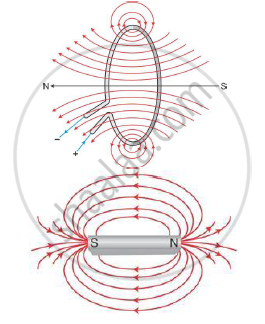Share

# Draw the Magnetic Field Lines Due to a Circular Loop - CBSE (Science) Class 12 - Physics

ConceptMagnetic Field Intensity Due to a Magnetic Dipole (Bar Magnet) Along Its Axis

#### Question

Draw the magnetic field lines due to a circular loop area vecA carrying current I. Show that it acts as a bar magnet of magnetic moment vecm = IvecA .

#### Solution

When a circular loop of area A carries a current I, the loop creates a magnetic field around it. The strength of the magnetic field created depends on the current through the conductor. At the centre of the loop, the magnetic field lines are perpendicular to the plane of the loop which provides the magnetic moment, m = IA. Hence, it behaves like a magnet. The comparison between the magnetic field lines around a current-carrying loop and a bar magnet shows that the two patterns are similar.If the current in the loop is in the anticlockwise direction, a North Pole is formed and if the current is in the clockwise direction, a South Pole is formed.

Is there an error in this question or solution?

#### APPEARS IN

Solution Draw the Magnetic Field Lines Due to a Circular Loop Concept: Magnetic Field Intensity Due to a Magnetic Dipole (Bar Magnet) Along Its Axis.
S